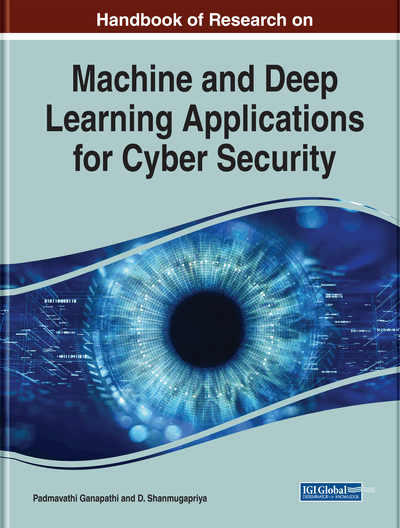# Variable Selection Method for Regression Models Using Computational Intelligence Techniques

Dhamodharavadhani S. (Periyar University, India) and Rathipriya R. (Periyar University, India)
Copyright: © 2020 |Pages: 21
DOI: 10.4018/978-1-5225-9611-0.ch019
OnDemand PDF Download:
Available
\$37.50
No Current Special Offers

## Abstract

Regression model (RM) is an important tool for modeling and analyzing data. It is one of the popular predictive modeling techniques which explore the relationship between a dependent (target) and independent (predictor) variables. The variable selection method is used to form a good and effective regression model. Many variable selection methods existing for regression model such as filter method, wrapper method, embedded methods, forward selection method, Backward Elimination methods, stepwise methods, and so on. In this chapter, computational intelligence-based variable selection method is discussed with respect to the regression model in cybersecurity. Generally, these regression models depend on the set of (predictor) variables. Therefore, variable selection methods are used to select the best subset of predictors from the entire set of variables. Genetic algorithm-based quick-reduct method is proposed to extract optimal predictor subset from the given data to form an optimal regression model.
Chapter Preview
Top

## Introduction

Describe Variable selection method plays a vital role to select the best subset of predictors. Variable selection method is the process of selection a subset of relevant predictors for fitting the model. In Regression model, variable selection is used to select the best subset of predictors to build the best regression model. Because redundant predictors are occurs in model that changes the behavior of effective predictors and also degree of freedom is misrepresented (Abraham A, 2003). There are many existing in the literature for regression model. Basically, three methods are used to select the variables for regression model. They are graphical represented in figure 1

The figure 2 describes the workflow of variable selection method. Generation Procedure implements a search method. This is used to generate subset of variables (Bjorvand, 1997). Evaluation Procedure is used to halt the process when an optimal subset is reached. Stopping Criterion is tested every iteration to determine whether the variable selection process should continue or not. If stopping condition has been satisfied, then the loop has been terminated. Validation procedure is used to validate the subset of variables (C. B. Lucasius, 1992).

### Filter Methods

Filter feature selection methods apply a statistical measure to allocate a value to each feature. The features are ranked based on the value and also selected or removed from the dataset. The methods are frequently univariate and reflect the feature independently, or with regard to the dependent variable.

### Wrapper Methods

A wrapper method is used to selection of a set of features as a search problem. sometimes different combinations are prepared, evaluated and compared to other combinations. A predictive model us used to evaluate a combination of features and assign a value based on model accuracy.

### Embedded Methods

Embedded methods study which features best donate to the accuracy of the model. The most common type of embedded feature selection methods are regularization methods. Regularization methods are also called penalization methods that introduce additional constraints into the optimization of a predictive algorithm (such as a regression algorithm) that bias the model toward lower complexity (fewer coefficients) (Airong Cai, 2009) (Bruce Ratner, 2010).

Feature selection is the method of reducing data dimension while doing predictive analysis. Various kinds of feature selection techniques are used in machine learning problem. The feature selection techniques simplify the machine learning models in order to make it easier to interpret by the researchers. Also, this technique reduces the problem of overfitting by enhancing the generalization in the model. Hence, it helps in better understanding of data, improves prediction performance, reducing the computational time as well as space which is required to run the algorithm (Airong Cai, 2009) (Bruce Ratner, 2010).

In this chapter, computational intelligence based variable selection method is introduced for regression model. It identify the optimal subset of predictors to build the effective regression model for better predicting accuracy.

## Key Terms in this Chapter

Swarm Intelligence: It refers to the peculiar group behavior followed by any of the biological systems.

Particle Swarm Optimization (PSO): Commonly used swarm intelligence algorithm devised based on the bird flocking behavior.

Genetic Algorithm (GA): It is an evolutionary algorithm used for solving various optimization problems.

## Complete Chapter List

Search this Book:
Reset